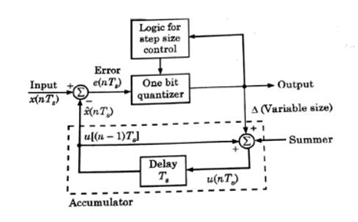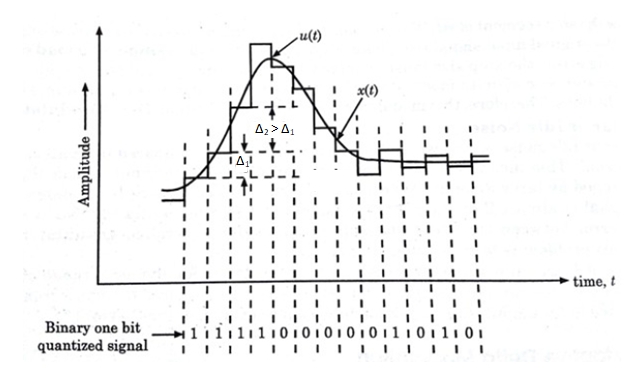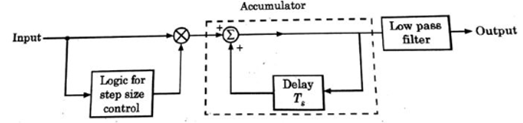# Explain Adaptive Delta Modulation and Compare its performance with Delta Modulation

### Reasons to use Adaptive Delta Modulation

In order to overcome the quantization errors due to slope overload and granular noise, the step size (Δ) is made adaptive to variations in the input signal x(t).

Particularly in the steep segment of the signal x(t), the step size is increased. And the step is decreased when the input is varying slowly.

The adaptive delta modulators can take continuous changes in step size or discrete changes in step size.

### Transmitter

Fig.1 shows the transmitter of an ADM.The logic for step size control is added in the diagram.

The step size increases or decreases according to a specified rule depending on one bit quantizer output.

For an example, if one bit quantizer output is high (i.e., 1), then step size may be doubled for next sample.

If one bit quantizer output is low, then step size may be reduced by one step.

Fig.2 shows the staircase waveforms of adaptive delta modulator and sequence of bits to be transmitted.Fig.2 : Waveform for adaptive delta modulationThe receiver has two portions. The first portion produces the step size from each incoming bit.Exactly the same process is followed as that in transmitter.

The previous input and present input decide the step size.

It is then applied to the second portion i.e., an accumulator which builds up staircase waveform.

The low pass filter then smoothens out the staircase waveform to reconstruct the original signal.